# 1. 采用思维导图描述本课程知识点脉络

1matlab与信号处理课程绪论
2Document目录
3实施编辑器
4App
5国庆放假
6Signal Analyser App
7Signal Processing Toolbox
8DSP System Toolbox
10Deep Learning 与 matlab
11复习串讲

# 2. MATLAB 编程

## 2.3. 实时脚本和函数

### 2.3.1. 什么是实时脚本或实时函数

MATLAB® 实时脚本和实时函数是交互式文档，它们在一个称为实时编辑器的环境中将 MATLAB 代码与格式化文本、方程和图像组合到一起。此外，实时脚本可存储输出，并将其显示在创建它的代码旁。

（1）直观浏览和分析问题

• 在单个交互式环境中编写、执行和测试代码
• 逐个运行代码或作为整个文件运行，查看结果和图形以及生成它们对应的源代码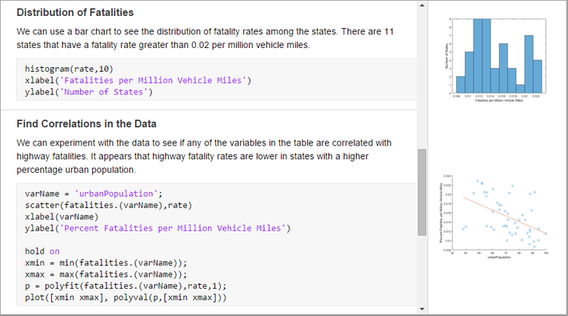（2）共享富文本格式的可执行记叙脚本

• 添加标题、题头和格式化文本以描述相应过程，并纳入方程、图像和超链接作为支撑材料
• 将您的记叙脚本另存问富文本格式的可执行文档，并与同事或 MATLAB 社区共享它们，或者将其转换为 HTML、PDF、Microsoft® Word 或 LaTeX 文档以供发布。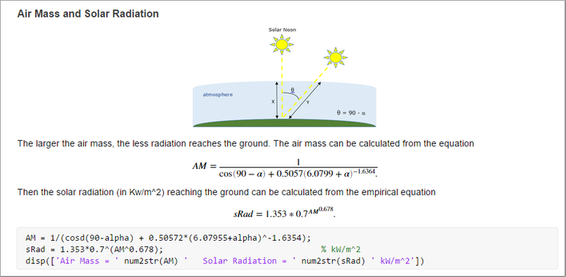（3）创建交互式教学课件

• 将代码和结果与格式化文本和数学方程结合使用。
• 创建分步式课件并逐步进行计算以说明教学主题。
• 随时修改代码以回答问题或探讨相关主题。
• 将课件作为交互式文档与学生共享或以硬拷贝形式共享，将部分完成的文件作为作业发给学生。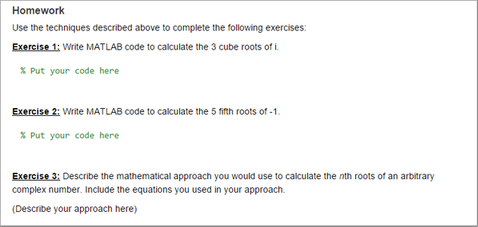### 2.3.2. 实时脚本和纯代码脚本的区别

（1）分类范围的区别：脚本是代码的一种。代码是指程序员在工作时使用编程软件敲出的字符、符号等元素组成的源文件，而脚本则是特指纯文字文本的程序，该程序同样是由程序源文件构成。因此代码的涵盖分类范围远远比脚本大。

（2）实现的结果区别：脚本就是写一个或多个工具使得一个或者一系列任务自动化。而代码的作用效果同样能带到脚本的作用，其次更是把多个源代码进程互相关联使用，而且还可以组成一个程序让计算机按照设计好的思路进行运行。

### 2.3.3. 共享实时脚本和函数

MATLAB 支持 R2016a 及更高版本中的实时脚本，以及 R2018a 及更高版本中的实时函数。

## 2.4. 类

MATLAB 类支持函数和运算符重载、对属性和方法的控制访问、引用和值语义以及事件和侦听程序。

### 2.4.1. 使用 MATLAB 进行面向对象的设计

#### 2.4.1.1. 为什么使用面向对象的设计

（1）MATLAB 程序的编程方式

• 确定要构建的系统或应用程序的组件
• 分析和识别模式，以确定哪些组件会重用或共享特征
• 基于相似性和差异性对组件进行分类

（2）何时创建面向对象的程序

• 约束赋给任何给定属性的数据值
• 仅在查询属性时计算属性值
• 查询或更改任何属性值时广播通知
• 限制对属性和方法的访问

1. 检查输入
2. 对第一个输入参数执行计算
3. 基于第二个输入参数转换步骤 2 的结果
4. 检查输出和返回值的有效性

Step 1
function checkInputs()
% actual implementation
end

Step 2
function results = computeOnFirstArg()
% specify syntax only
end

Step 3
function transformResults()
% actual implementation
end

Step 4
function out = checkOutputs()
% actual implementation
end


• 确定特定目标的需求
• 将需求作为接口类编写到您的程序中

• 对象提供隐藏实现细节的接口。
• 对象实施控制对象交互方式的规则。

• 公共 - 任何代码都可以访问此特定属性或调用此方法。
• 受保护 - 只有此对象的方法和从此对象的类派生的对象的方法才能访问此属性或调用此方法。
• 私有 - 只有对象自己的方法才能访问此属性或调用此方法。

### 2.4.2. 什么是 System Object

System object™ 是一种 MATLAB® 类，具有用于算法建模的特定方法和属性。System object 适用于为动态系统建模和处理流式数据。您可以使用系统工具箱产品中包含的预定义 System object，也可以定义自己的 System object。

System object™ 是一种专用的 MATLAB® 对象。许多工具箱中都包含 System object。System object 专为实现和仿真输入随时间变化的动态系统而设计。许多信号处理、通信和控制系统都是动态的。在动态系统中，输出信号的值同时取决于输入信号的瞬时值以及系统的过往行为。System object 使用内部状态来存储下一个计算步骤中使用的系统过往行为。因此，System object 非常适用于分段处理大型数据流的迭代计算，例如视频和音频处理系统。这种处理流化数据的功能具有不必在内存中保存大量数据的优点。采用流化数据，您还可以使用可高效利用循环的简化程序。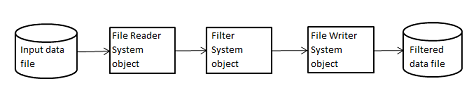（1）运行 System Object

（2）System object 函数

#### 2.4.2.2. System Object 与 MATLAB 函数

（1）System Object 与 MATLAB 函数

（2）使用仅包含 MATLAB 函数的代码处理音频数据

fname = 'speech_dft_8kHz.wav';


audioInfo = audioinfo(fname);
maxSamples = audioInfo.TotalSamples;
fs = audioInfo.SampleRate;


b = fir1(160,.15);


z = zeros(1,numel(b)-1);


frameSize = 1024;
nIdx = 1;


while nIdx <= maxSamples(1)-frameSize+1
audio = audioread(fname,[nIdx nIdx+frameSize-1]);
[y,z] = filter(b,1,audio,z);
sound(y,fs);
nIdx = nIdx+frameSize;
end


（3）使用 System object 处理音频数据

fname = "speech_dft_8kHz.wav";


audioIn = dsp.AudioFileReader(fname,'OutputDataType','single');


filtLP = dsp.FIRFilter('Numerator',fir1(160,.15));


audioOut = audioDeviceWriter('SampleRate',audioIn.SampleRate);


while ~isDone(audioIn)
audio = audioIn();    % Read audio source file
y = filtLP(audio);   % Filter the data
audioOut(y);         % Play the filtered data
end


# 3. 描述 MATLAB 产品架构和帮助文档架构

## 3.1. matlab 产品架构

MATLAB是MATLAB产品家族的计算核心与基础，是集高性能数值计算与数据可视化于一体的高效编程语言。围绕着MATLAB这个计算核心，形成了诸多针对不同应用领域的算法程序包，被称为专用工具箱（Toolbox），这些工具箱的列表以及每个工具箱的使用详见MATLAB在线帮助文档。MATLAB本身所提供的工具箱大概有40多个，另外还有其他公司或研究单位开发提供的工具箱，这些工具箱的总数已有100多个，而且新的工具箱还在不断增加。如果你有特别的应用领域，可以首先到网上查找是否已有相关的工具箱，很可能已有人将你要做的应用程序作成工具箱了。

MATLAB Compiler这种编译器可以将MATLAB程序文件编译生成标准的C/C++语言文件，而生成的标准的C/C++文件可以被任何一种C/C++编译器编译生成函数库或可执行文件，以提高程序的运行效率。

## 3.2. 帮助文档架构

### 3.2.1. matlab 帮助文档介绍

• 使用doc命令在单独的窗口中打开看书文档
• 使用help命令可在命令行窗口中查看相应函数的简明文档
• 在键入函数输入参数的左括号之后暂停，此时命令行窗口中会显示相应函数的提示（函数文档的语法部分）。如mean(

# 5. Signal Processing Toolbox

Signal Processing Toolbox™ 提供了一些函数和 App，用来分析、预处理及提取均匀和非均匀采样信号的特征。该工具箱包含可用于滤波器设计和分析、重新采样、平滑处理、去趋势和功率谱估计的工具。该工具箱还提供了提取特征（如变化点和包络）、寻找波峰和信号模式、量化信号相似性以及执行 SNR 和失真等测量的功能。您还可以对振动信号执行模态和阶次分析。

1. 信号分析和可视化
使用 Signal Analyzer 来可视化、预处理和探查信号
2. 信号生成和预处理
对信号进行创建、重新采样、平滑、去噪和去趋势处理
3. 测量和特征提取
波峰、信号统计、脉冲和瞬态指标、功率、带宽、失真
4. 变换、相关性和建模
互相关、自相关、傅里叶、DCT、Hilbert、Goertzel、参数化建模、线性预测编码
5. 数字和模拟滤波器
FIR 和 IIR、单速率和多速率滤波器设计、分析和实现
6. 频谱分析
功率谱、相干性、窗口
7. 时域分析
频谱图、交叉频谱图、同步压缩、重排、Wigner-Ville、Hilbert-Huang、kurtogram
8. 振动分析
阶数分析、时间同步平均、包络频谱、模态分析、雨流计数
9. 信号的及去学习和深度学习延伸
信号标注、特征工程、数据集生成

## 5.1. 信号分析和可视化

Signal Analyzer App 是一款交互式工具，用于在时域、频域和时频域中可视化、测量、分析和比较信号。通过该 App 可同时和在同一视图中处理不同持续时间的许多信号。

[外链图片转存失败,源站可能有防盗链机制,建议将图片保存下来直接上传(img-Ncwxuera-1606572446391)(https://ww2.mathworks.cn/help/signal/ug/using-signal-analyzer-app.html)]

### 5.1.1. 使用 Signal Analyzer App

（1）APP 工作流

1. 选择要分析的信号 - 选择 MATLAB® 工作区中可用的任何信号。该 App 接受具有固有时间信息的数值数组和信号，例如 MATLABtimetable 数组、timeseries 对象和 labeledSignalSet 对象。有关详细信息，请参阅Data Types Supported by Signal Analyzer。

2. Preprocess Signals - 低通、高通、带通或带阻滤波器信号。去趋势并计算信号包络。使用移动平均值、回归、Savitzky-Golay 滤波器或其他方法对信号进行平滑处理。更改信号的采样率或将非均匀采样的信号插值到均匀网格上。使用您自己的自定义函数预处理信号。生成 MATLAB 函数来自动执行预处理操作。

3. 探查信号 - 使用采样率、数值向量、duration 数组或 MATLAB 表达式向信号添加时间信息。绘制、测量和比较数据、其频谱、频谱图或尺度图。寻找时域、频域和时频域中的特性和模式。计算持久性频谱以分析偶发信号，并使用重排来锐化频谱图估计。从信号中提取关注的区域。

4. 共享分析 - 将显示内容作为图像从 App 复制到剪贴板。将信号导出到 MATLAB 工作区或将其保存到 MAT 文件。生成 MATLAB 脚本，以自动计算功率谱、频谱图或持久性频谱估计，并自动提取关注区域。保存 Signal Analyzer 会话，以便以后或在另一台机器上继续分析。

（2）频谱和时域分析

## 5.2. 信号生成和预处理

Signal Processing Toolbox™ 提供的函数可让您对信号进行去噪、平滑和去趋势处理，为进一步分析做好准备。从数据中去除噪声、离群值和乱真内容。增强信号以对其可视化并发现模式。更改信号的采样率，或者使不规则采样信号或带缺失数据信号的采样率趋于恒定。为仿真和算法测试生成脉冲信号和 chirp 等合成信号。

some theme

1. Signal Generation and Visualization
2. 将开始时间不同的信号对齐
3. Find a Signal in a Measurement
4. Reconstruct a Signal from Irregularly Sampled Data

## 5.3. 测量和特征提取

Signal Processing Toolbox™ 提供的函数可用于测量信号的常见不同特征。定位信号波峰并确定其高度、宽度和与邻点的距离。测量时域特征，如峰间幅值和信号包络。测量脉冲指标，如过冲和占空比。

some theme

• Peak Analysis
• Detecting Outbreaks and Significant Changes in Signals
• Finding a Signal in Data
• Measurement of Pulse and Transition Characteristics
• Analyzing Harmonic Distortion
• Extracting Classification Features from Physiological Signals

## 5.4. 变换、相关性和建模

Signal Processing Toolbox™ 提供了用于计算信号的相关性、卷积和变换的函数。使用快速傅里叶变换将数据分解成若干频率分量。通过使用传递函数对信号求卷积对信号进行滤波。使用相关性量化信号相似性。使用离散余弦变换来压缩数据。

• 离散傅里叶变换
• 使用自相关求周期性
• 使用 FFT 获得功率频谱密度估计
• 线性和循环卷积

## 5.5. 数字和模拟滤波器

FIR 和 IIR、单速率和多速率滤波器设计、分析和实现

Signal Processing Toolbox™ 提供的函数和 App 可用于设计、分析和实现各种数字 FIR 和 IIR 滤波器，如低通滤波器、高通滤波器和带阻滤波器。可视化幅值、相位、群延迟、脉冲和阶跃响应。检查滤波器极点和零点。通过测试稳定性和相位线性来计算滤波器性能。对数据应用滤波器，并使用零相位滤波消除延迟和相位失真。

Signal Processing Toolbox 还提供一些函数，用于设计和分析模拟滤波器，包括 Butterworth、Chebyshev、Bessel 和椭圆设计。使用脉冲不变性和双线性变换等离散化方法执行模数滤波器转换。

FIR、IIR、加窗、等波纹、最小二乘、Butterworth、Chebyshev、椭圆、脉冲整形

Bessel、Butterworth、Chebyshev、椭圆、双线性变换、分析函数

## 5.6. 频谱分析

Signal Processing Toolbox™ 提供一系列频谱分析函数和 App，用于表征信号的频谱。基于 FFT 的非参数化方法，如 Welch 的方法或周期图，对输入数据不做任何假设，可用于任何类型的信号。参数化方法和子空间方法，如 Burg 法、协方差法和 MUSIC 法，结合信号的先验知识，可以产生更准确的频谱估计。

Burg、Yule-Walker、协方差和修正协方差方法

Hamming、Blackman、Bartlett、Chebyshev、Taylor、Kaiser

## 5.7. 时频分析

Signal Processing Toolbox™ 提供的函数和 App 可用于可视化和比较非平稳信号的时频内容。计算短时傅里叶变换及其逆变换。使用重排或傅里叶同步压缩获得清晰的频谱估计。绘制交叉频谱图、Wigner-Ville 分布和持久性频谱。提取并跟踪时频脊。估计瞬时频率、谱峭度和频谱熵。使用经验模态分解和 Hilbert-Huang 变换执行数据自适应时频分析。

## 5.8. 振动分析

Signal Processing Toolbox™ 提供的函数可用于研究和表征机械系统中的振动。使用阶数分析来分析和可视化旋转机械中出现的频谱内容。跟踪和提取阶数及其时域波形。将信号的平均频谱估计为阶数的函数。通过估计频率-响应函数、固有频率、阻尼比和模态形状来执行试验模态分析。绘制稳定图。使用时间同步平均法连贯地去除噪声，并使用包络频谱分析磨损。为疲劳分析生成高循环雨流计数。

## 5.9. 信号的机器学习和深度学习延伸

Signal Processing Toolbox™ 为机器学习和深度学习工作流提供执行信号标注、特征工程和数据集生成的功能。

# 6. DSP System Toolbox

DSP System Toolbox™ 提供多种算法、App 和示波器，用于在 MATLAB® 和 Simulink® 中设计、仿真和分析信号处理系统。您可以为通信、雷达、音频、医疗设备、IoT 和其它应用进行实时 DSP 系统建模。

DSP System Toolbox 支持您设计和分析 FIR、IIR、多速率、多级和自适应滤波器。您可以从变量、数据文件和网络设备流式传输信号以进行系统开发和验证。时域示波器、频谱分析器和逻辑分析器支持您对流信号进行动态可视化和测量。在桌面原型构建和嵌入式处理器（包括 ARM® Cortex® 架构）部署方面，该系统工具箱支持 C/C++ 代码生成。它还支持从滤波器、FFT、IFFT 和其他算法进行位真定点建模和 HDL 代码生成。

1. Signal Generation, Manipulation, and Analysis
Create, import, export, display, and manage signals

2. Filter Design and Analysis
FIR, IIR, frequency transformations

3. Filter Implementation
Single-rate, multirate, and adaptive filters

4. Transforms and Spectral Analysis
FFT, DCT, spectral analysis, linear prediction

5. Statistics and Linear Algebra
Measurements, statistics, matrix math, linear algebra

6. Fixed-Point Design
Floating-point to fixed-point conversion, fixed-point algorithm design

7. Code Generation
Simulation acceleration, code generation, optimization for ARM Cortex-M Processors and ARM Cortex-A Processors

8. Applications
Simulate radar, communication, and biomedical systems

## 6.1. 信号的产生、处理和分析

DSP System Toolbox™ 提供了使用 MATLAB 或 Simulink 在时间和频率域分析、测量和可视化信号的工具。您可以生成和传输信号，对这些信号执行操作，并实时可视化这些变化。要动态地可视化信号，可以使用频谱分析仪、时间范围、数组图和逻辑分析器块或系统对象。

Signal Operations 信号操作
Delay, interpolate, reorder, resample, window 延迟，插值，重新排序，重采样，窗口

Signal Generation 信号产生
Create signals 创建信号

Signal Input and Output 信号输入和输出
Interface with external signals 与外部信号接口

Scopes and Data Logging 范围和数据记录
Display and record data 显示和记录数据

Buffers, Switches, and Counters 缓冲器、开关和计数器
Change sample rate or frame rate of signals, count events, detect edges, perform actions when events occur 改变采样率或帧速率的信号，计数事件，检测边缘，执行行动时，事件发生

Signal Attributes and Indexing 信号属性和索引
Inspect and modify data and signal attributes and select data subsets 检查和修改数据和信号属性，并选择数据子集

## 6.2. Filter Design and Analysis

FIR, IIR, frequency transformations

You can design and analyze a variety of digital FIR and IIR filters using DSP System Toolbox™ functions and apps. Some of these filters include advanced filters such as Nyquist filters, halfband filters, advanced equiripple filters, and quasi-linear phase IIR filters.

The design techniques compute the filter coefficients of based on the specifications. The analysis techniques help you validate the specifications of the designed filter. Analysis techniques include plotting the frequency response of the filter, finding the group delay of the filter, or determining if the filter is stable.

Filter design and analysis are complementary and iterative. After you design a filter, analysis tools help you determine if the filter meets the required specifications. The toolbox provides design and analysis apps such as filterBuilder and fvtool. To learn how to use filterBuilder, see Filter Builder Design Process.

You can also transform filters from one form to another form using functions such as firlp2hp, iirlp2bs, iirlp2bpc.

Filter Design
FIR, IIR

Filter Analysis
Analyze filters and filter objects

Frequency Transformations
Transform filters to other forms, use features in a filter to develop another filter

## 6.3. Filter Implementation

Single-rate, multirate, and adaptive filters

DSP System Toolbox™ provides filter blocks and System objects that enable you to filter a streaming signal in MATLAB® and Simulink®. In addition to the conventional FIR and IIR filters, this toolbox also provides specialized filters such as multirate multistage filters, wave digital filters, and Farrow filters.

Single-Rate Filters 单速率过滤器
FIR, IIR, overlap-add FIR, biquad, lattice, Farrow

Multirate and Multistage Filters 多速率多级滤波器
Decimation, interpolation, rate conversion 抽取，插值，速率转换

LMS, RLS, frequency domain filters, affine projection filter, adaptive lattice filter LMS，RLS，频域滤波器，仿射投影滤波器，自适应格型滤波器

## 6.4. Transforms and Spectral Analysis

FFT, DCT, spectral analysis, linear prediction

The frequency-domain representation of a signal reveals important signal characteristics that are difficult to analyze in the time domain. Spectral analysis lets you characterize the frequency content of a signal. The FFT and IFFT System objects and blocks in DSP System Toolbox™ enable you to convert a streaming time-domain signal into the frequency-domain, and vice versa. To compute the spectral estimate of the signal, use the dsp.SpectrumEstimator System object™ in MATLAB® and the Spectrum Estimator block in Simulink®. You can visualize the spectral estimate using the spectrum analyzer System object and block.

The spectrum analyzer in DSP System Toolbox uses the Welch’s method of averaging modified periodogram and the filter bank method. Both these methods are FFT-based spectral estimation methods that make no assumptions about the input data and can be used with any kind of signal. For more information on the algorithm the spectrum analyzer uses, see Spectral Analysis.

Transforms
DCT, FFT, HDL FFT, HDL IFFT, IDCT, IFFT

Linear Prediction
Convert between linear predictive coefficients (LPC) and cepstral coefficients, LSF, LSP, and RC

Spectral Analysis
Parametric and nonparametric methods

## 6.5. 统计和线性代数

DSP System Toolbox™ 提供了用于测量流信号统计的功能，例如 MATLAB® 和 Simulink® 中的均值，中值，RMS 和峰峰值。 您可以对这些信号执行多种操作，例如沿指定尺寸添加信号值并将信号值转换为 dB 标度。

DSP System Toolbox 系统线性代数模块使您可以处理矩阵。 例如，要通过Levinson-Durbin 和 Cholesky 方法求解一组线性方程，请使用 Levinson-Durbin 和Cholesky Solver 块。 这些技术通常用于诸如 Yule-Walker AR 问题和线性预测编码之类的应用中。 要将方矩阵分解为上下分量，请使用 LDL分解，LU 分解和 QR 分解等方法。

DSP System Toolbox™ provides functionality for measuring streaming signal statistics such as mean, median, RMS, and peak-to-peak values in MATLAB® and Simulink®. You can perform several operations on these signals, such as adding the signal values along a specified dimension and converting the signal values into a dB scale.

DSP System Toolbox system linear algebra blocks enable you to work with matrices. For example, to solve a set of linear equations through Levinson-Durbin and Cholesky methods, use the Levinson-Durbin and Cholesky Solver blocks. These techniques are commonly used in applications such as the Yule-Walker AR problem and linear predictive coding. To factorize a square matrix into upper and lower components, use methods such as LDL factorization, LU factorization, and the QR factorization.

Measurements and Statistics
Bistate waveform measurements, variance, histogram, autocorrelation

Array and Matrix Mathematics
Array and matrix operations, polynomial fitting

Linear Algebra
Solve linear equations, factor and invert matrices

## 6.6. Fixed-Point Design

Floating-point to fixed-point conversion, fixed-point algorithm design

To model fixed-point signal processing algorithms and analyze the effects of quantization on system behavior and performance, you can use the DSP System Toolbox™ and Fixed-Point Designer™ functionality.

Perform design tradeoff analyses and optimizations on these algorithms by simulating them with different word lengths, scaling, overflow handling, and rounding method choices before you commit to hardware.

Fixed-Point Overview 定点概览
Basics of fixed-point concepts and terminology 定点概念和术语基础

Fixed-Point Filters 定点滤波器
Fixed-point filter design 定点滤波器设计

Floating-Point to Fixed-Point Conversion 浮点到定点的转换
Algorithm and model conversion 算法与模型转换

Quantizers 量化器
Encoders, decoders, scalar and vector quantizers 编码器，解码器，标量和矢量量化器

## 6.7. Simulink 中的信号处理和线性代数模块

Simulink 包含 DSP System Toolbox™ 信号处理算法模块库，涉及滤波器、变换和线性代数。这些模块将流输入信号处理为单个采样或采样集合（又称“帧”）。基于采样的处理模式支持需要标量处理的低延迟处理和应用。基于帧的处理模式支持以延迟换取更高吞吐量。系统工具箱可同时支持基于采样和基于帧的处理模式。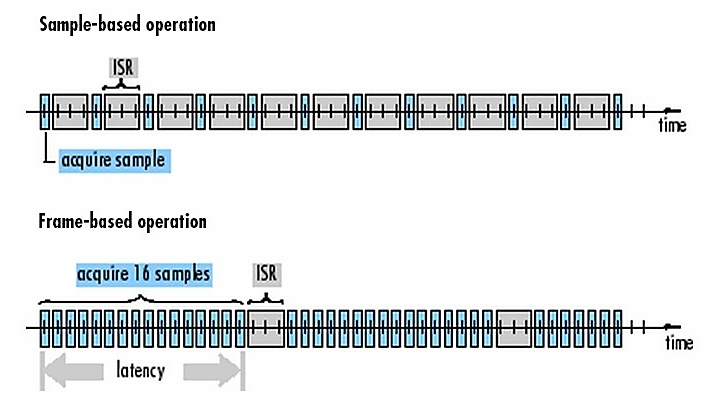### 6.7.1. 针对 DSP 系统设计、实现和测试的信号处理模块

Simulink 信号处理模块支持双精度和单精度浮点数据类型和整数数据类型，与 Fixed-Point Designer 配合使用时，还支持定点数据类型。

DSP System Toolbox 中的信号处理模块包括：

• 信号变换，如快速傅立叶变换 (FFT)、离散余弦变换 (DCT)、短时傅立叶变换 (STFT) 和离散小波变换 (DWT)
• FIR、IIR 和模拟滤波器的滤波器设计和实现
• 用于采样率转换的多速率和多级滤波器，如CIC、半带、多相和 Farrow
• 用于频谱估计、均衡和噪声抑制的统计和自适应信号处理技术
• 信号操作和测量，如卷积、加窗、填充、延迟、峰值查找和过零
• 使用时域示波器、频谱分析器等工具进行的流信号可视化和测量
• 信号管理方法，如缓冲、索引、切换、堆栈和队列
• 接收端和发送端，如线性调频和有色噪声生成器、NCO、UDP 接收机和发射机等
• 数值线性代数例程，包括线性方程组求解器、矩阵分解和矩阵求逆

Simulink 中的 DSP System Toolbox 信号处理模块库（上），以及线性方程组求解器（左下）和变换（右下）的扩展视图。

## 6.8. MATLAB 中的流信号处理

DSP System Toolbox 为 MATLAB 中的流信号处理提供框架。该系统工具箱带有一个针对流信号处理进行优化的信号处理算法库，涉及单速率和多速率滤波器、自适应滤波器和 FFT。该系统工具箱是您设计、仿真和部署信号处理解决方案的理想选择，适用于音频、生物医学、通信、控制、地震、传感器和语音等多种应用领域。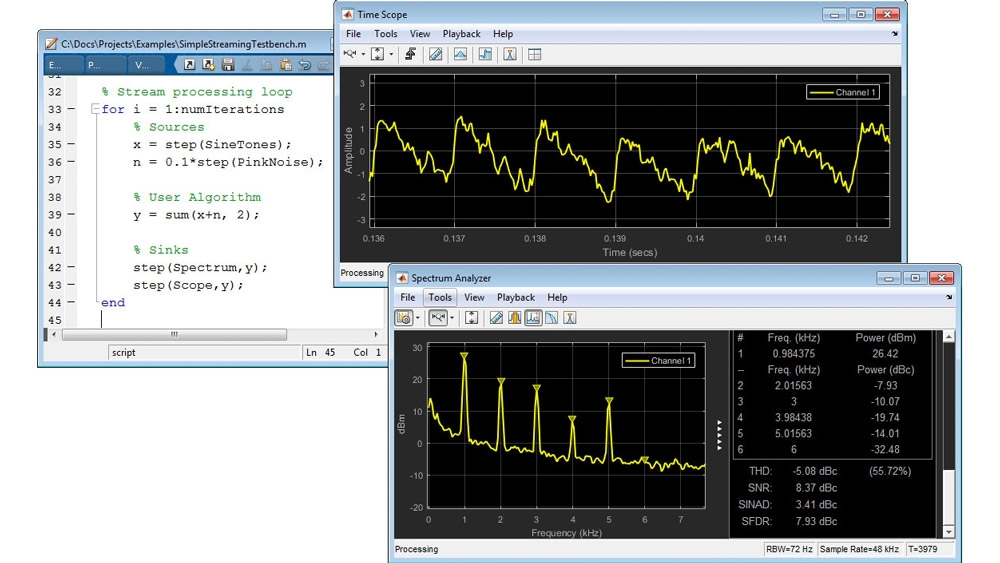MATLAB 代码实现基本流循环（左）。时域示波器（右上）和频谱分析器（右下）在实时信号生成和处理的同时对其进行可视化和测量。

### 6.8.1. DSP 系统设计、实现和测试的算法库

DSP System Toolbox 提供超过 350 种针对流系统的设计、实现和验证进行优化的算法，无论作为 MATLAB 函数实现还是作为 MATLAB System object实现。这些算法均支持双精度和单精度浮点数据类型。大多数算法还支持整数数据类型，也支持定点数据类型（需要 Fixed-Point Designer™）。

• 信号变换，如快速傅立叶变换 (FFT)、离散余弦变换 (DCT)
• 用于数字 FIR 和 IIR 滤波器的设计和实现方法
• 用于采样率转换的多速率和多级滤波器，如 FIR 和 IIR 半带、多相滤波器、CIC 滤波器和 Farrow 滤波器
• 用于频谱估计、均衡和噪声抑制的统计和自适应信号处理方法
• 信号操作和测量，如卷积、加窗、填充、建模延迟、峰值查找和可变分数延迟
• 运行时信号可视化，借助时域示波器、频谱分析器和逻辑分析器实现

MATLAB 中提供的部分信号处理算法，可由命令行帮助显示，也可通过 Tab 键自动填充查找。

## 6.9. 单速率和多速率 FIR 和 IIR 滤波器设计以及自适应滤波器

DSP System Toolbox 提供了大量 FIR、IIR、多级、多速率和自适应滤波器的滤波器设计和实现算法。您可以设计低通、高通、带通、带阻以及其他响应类型的滤波器。您还可以采用直接型 FIR、叠加 FIR、IIR 二阶节（双二阶）、级联全通和格型结构等滤波器结构来实现这些滤波器。

• 高级等波纹 FIR 滤波器，包括最小阶、约束波纹和最小相位设计
• Nyquist、FIR 半带和 IIR 多相滤波器，提供线性相位、最小相位和准线性相位半带设计以及等波纹、斜向频率阻带和窗函数方法
• CIC 插值器和抽取器滤波器，用于软件定义无线电和 Sigma-Delta 转换器中的无乘法实现
• 优化的多级设计，可通过优化级联的级数实现最低的计算复杂度
• 分数延迟滤波器，包括基于 Farrow 滤波器结构的实现，适用于可调滤波应用
• 具有任意群延迟的全通 IIR 滤波器，可以补偿其他 IIR 滤波器的群延迟，从而获得近似线性的通带相位响应
• 格波数字 IIR 滤波器，具有稳健的实现
• 任意幅值和相位的 FIR 和 IIR 滤波器，支持各种滤波器设定的设计

### 6.9.1. 自适应滤波器

DSP System Toolbox 提供多种方法，支持在 MATLAB 和 Simulink 中进行自适应滤波。这些技术广泛运用于系统识别、频谱估计、均衡和噪声抑制等应用场合。此类自适应滤波器包括基于 LMS、基于 RLS、仿射投影、快速横向、频域、基于格型和卡尔曼滤波器。该系统工具箱还包含用于分析这些自适应滤波器的算法，包括系数跟踪、学习曲线和收敛。

### 6.9.2. 多速率和多级滤波器和分析

DSP System Toolbox 提供多速率滤波器的设计和实现，包括多相插值器、抽取器、采样率转换器、FIR 半带和 IIR 半带、Farrow 滤波器以及 CIC 滤波器和补偿器，并且支持多级设计方法。该系统工具箱还提供专用分析函数，用于估计多速率和多级滤波器的计算复杂度。

## 6.10. 信号示波器、分析器和测量工具

DSP System Toolbox 提供示波器和数据记录功能，可用于 MATLAB 和 Simulink 中流信号的时域或频域可视化、测量和分析。这些示波器的测量和统计功能与行业标准示波器和频谱分析器类似，符合用户习惯。

DSP System Toolbox 还提供一系列可视化工具，可用于显示和测量各种信号或数据，包括实数值或复数值数据、向量、数组和任意数据类型（包括定点、双精度或用户定义的数据输入序列）的帧。其中一些可视化工具提供流数据或信号的三维显示，以便您持续分析数据直至仿真停止。

# 7. Signal Processing Toolbox 和 DSP System Toolbox 的联系和区别

## 7.1. 联系

• Signal Processing Toolbox 和 DSP System Toolbox 均是利用基本的信号与系统知识，可以对信号做一些基本的分析和处理。
• 均可产生一些特定的信号，如 chirp 信号，脉冲信号等。
• 均可设计数字滤波器如 FIR, IIR 滤波器
• 均可对信号在时域和频域进行转化并分析
• 等等

## 7.2. 区别

• Signal Processing Toolbox 侧重于对信号进行分析，提取信号的各种特征；而 DSP System Toolbox 侧重于对信号进行处理。
• Signal Processing Toolbox 提供了很多函数，侧重于面向过程编程，而DSP System Toolbox 更侧重于面向对象编程。
• DSP System Toolbox 提供了很多 System Object 和 Blockset，和Simulink 联系很紧密。可以在 Simulink 利用 DSP System Toolbox所提供的各种 Blockset 进行建模和仿真，并利用示波器等达到数据的可视化。
• DSP System Toolbox 提供了很多算法库可以对流信号进行处理，而 SPToolbox 确没有提供这样的算法库。
• 等等，总之，DSP System Toolbox 更加强大，更加侧重于工程应用，
• SPToolbox 侧重于对信号进行分析，并可以对信号进行一些简单的处理如去趋势，平滑去噪等。

## 8.1. 应用领域

• 一般应用领域
说明一般应用的示例模型
• 汽车应用领域
使用 Simulink® 和其他 MathWorks® 产品对汽车系统进行建模和仿真
• 航空应用领域
使用 Simulink 和 Aerospace Blockset™ 软件进行航空航天系统建模
• 工业自动化应用领域
说明工业自动化应用的示例模型
• 信号处理
使用 DSP System Toolbox™ 软件对信号处理和通信系统建模
• 物理建模
使用 Simscape™ 软件进行物理系统建模
• 复杂逻辑
使用 Stateflow® 图为复杂逻辑建模
• 离散事件仿真
进行离散事件系统建模和仿真
• 系统工程
使用 System Composer™ 软件设计和分析系统体系结构
• 大型建模
适用于大型模型和多用户开发团队的模型架构

## 8.2. Simulink 环境基础知识

### 8.2.1. Simulink 概念

#### 8.2.1.1. Systems and Models

Mathematical description of systems and models for those systems

The concept in this topic provide a common language for using Simulink software tools.

（1）System
A system is a group of interdependent physical and functional parts with measurable characteristics that change over time.

For example, a vehicle is a system with multiple parts. Measurable characteristics include the linear speed of the vehicle and the rotational speed of the wheels.

（2）System Component
A system component is part of a system that interacts with the other parts of the system. The interactions between components define the structure and behavior of the system.

For example, a cruise control module is a system component in a vehicle system. A microcontroller and the hardware associated with it define the structure while a software algorithm to control speed defines the behavior.

（3）Model
A model is a mathematical description of a system derived either from physical laws or experimental data. The description typically uses a set of variables with a set of differential and difference equations that define the relationships between the variables.

In the following example for a vehicle, u ( t ) u(t) is the force (N) moving a vehicle forward, v ( t ) v(t) is the velocity ( m / s m/s ), b is a drag coefficient ( N ׂ ׂ ⋅ s / m Nׂׂ·s/m ), and m m is the mass of the vehicle (kg).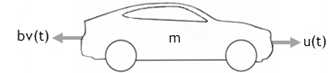The vehicle is a continuous system. For continuous systems, differential equations describe the rate of change for variables with the equations defined for all values of time. The velocity of the vehicle v(t) and its acceleration v'(t) are defined with the following first order differential equation.

m v ′ ( t ) + b v ( t ) = u ( t ) mv'(t) + bv(t) = u(t)

You can create a Simulink model for this equation by adding blocks, specifying block behavior, and using signal lines to connect the blocks to each other. The following Simulink block diagram implements the differential equation.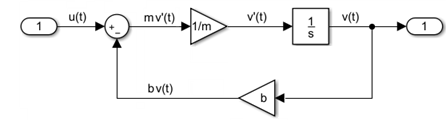（4）Model Component
A model component is part of a model that interacts with the other parts through an interface of inputs and outputs. Simulink implements model components using Subsystem and Model blocks. A Model block references another Simulink model saved in a separate file.

In the following example, the control model was saved in the Simulink model file control_model.slx, and then referenced from a Model block in a second Simulink model. A Subsystem block was added for modeling the vehicle mechanics.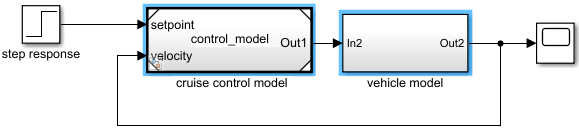Typically, controllers are built with discrete systems using a computer to implement the control algorithm. For discrete systems, difference equations describe the rate of change for variables defined only at specific times. For example, the control signal for a simple discrete PI (proportional–integral) controller can be defined with the following difference equation.

P I [ n ] = e [ n ] K p + ( e [ n ] + i n t e g r a l [ n − 1 ] ) K i PI[n] = e[n]Kp + (e[n]+integral[n-1])Ki

Where e[n] is the error between a signal whose value is controlled (velocity) and the specified value (set velocity), Kp is the proportion constant, Ki is the integration constant, and n is the time step.

The following Simulink block diagram implements the difference equation.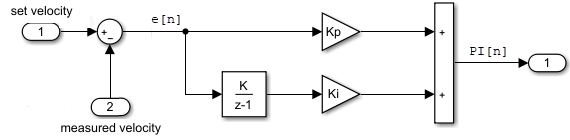#### 8.2.1.2. Simulink Models

A model is an abstract and simplified description of a system using mathematical equations and diagrams. The modeling concepts in this topic provide context for understanding the process of mathematically describing a system with Simulink® software tools.

（1）Block Diagram
A block diagram is a visual representation of a model in the Simulink Editor. The editor allows you to add blocks selected from block libraries representing elementary model components. Elementary components include integrator, gain, and sum blocks. Blocks are connected to each other with signal and event lines to visually construct the model equations.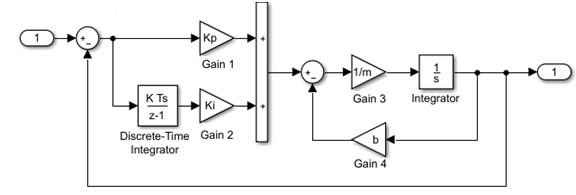Block Diagram Semantics
A classic block diagram of a system is drawn graphically with blocks and lines. The history of these block diagrams is derived from engineering areas such as Feedback Control Theory and Signal Processing. A block within a block diagram defines a model in itself. The relationships between elementary models are represented by signal lines connecting the blocks. The blocks and lines in a block diagram collectively describe the overall model of a system.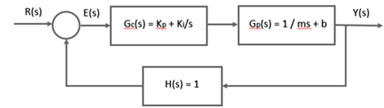Simulink extends classic block diagrams by:

• Adding a set of equations (block methods) to each block that defines the time-based relationships between the input signals, output signals, and block state variables.

• Adding parameters to each block that specify the coefficients from the model equations.

• Providing an engine for numerical solution of the block diagram by evaluating the relationships over time, where time starts at a user-specified “start time” and ends at a user-specified “stop time.”

（2）Blocks

A block is a basic modeling construct of the Simulink Editor. Add blocks from the built-in Simulink libraries to perform specific operations. You can also create custom blocks. Some blocks have input signals, output signals, and states. Most blocks have parameters that you use to specify block behavior. Whether a block has parameters and the nature of those parameters is specific to each block.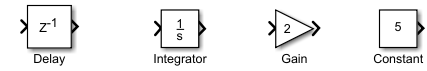Each block represents a set of equations for the Simulink engine. The equations are represented as block methods. The blocks shown above have the following block methods.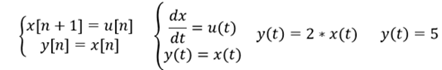Block methods are evaluated during the simulation of a block diagram. The evaluation of the block methods is performed within a simulation loop, where each cycle through the simulation loop represents the evaluation of the block diagram at a given point in time.

Virtual Blocks

Virtual blocks organize and provide graphical hierarchy in a model, but they do not affect the simulation result. During model simulation, Simulink expands the blocks in place before execution, a process known as flattening. This expansion is similar to the way macros work in a programming language such as C or C++.

Simulink defines the following virtual blocks:

• Virtual Subsystem – Use a virtual subsystem to encapsulate related and functional parts within a larger model. A Virtual Subsystem block has the check box for the parameter Treat as atomic unit cleared.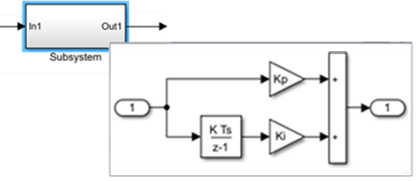• Inport and Outport – Use port blocks to move data (signals) and events (function calls) from outside a Subsystem block or referenced Model block to within the block, and vice versa.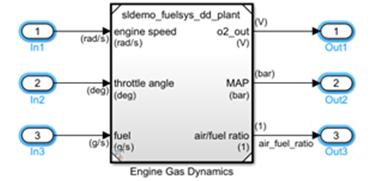• Bus Creator, Bus Assignment, Bus Selector – Bus blocks combine signals into a virtual bus and manage the routing of signals around a complex block diagram. Virtual buses are a graphical convenience and do not change the behavior of the model.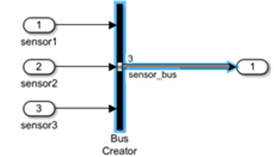Nonvirtual Blocks
Nonvirtual blocks provide execution control and graphical hierarchy in a model.

（3）Lines
A line is a basic modeling construct of the Simulink Editor. Lines connect the output ports of blocks with the input ports of other blocks.

（4）Data

Data includes the parameter and input signal values that Simulink uses to produce the output values resulting from simulating the behavior of a model.

（5）Parameters
Parameters are Simulink model characteristics that affect the simulation and code generation result.

（6）Properties
Properties are Simulink model characteristics that generally do not affect the simulation result. To specify properties, in the Modeling tab, under Design, click Property Inspector.

（7）State variables
The state of a model is defined by the values of its state variables. State variables are the set of variables whose values at time zero, along with the values of model inputs and the model equations, can determine the behavior of a model during a simulation. Examples of state variables include motor position and velocity, inductor current, capacitor voltage, solution temperature, and gas pressure.

If the current output value of a block is a function of the previous output value, the block defines a state variable that needs to be saved between time steps. Computing a block output hence entails saving the value of the state variable at the current time step for use in computing the output at a subsequent time step.

#### 8.2.1.3. Simulink Simulation

Simulating the model of a dynamic system allows you to gain insight about the behavior of a proposed system design without the time consuming process of actually building the system. The concepts in this topic provide a context for understanding how to control a model simulation with Simulink® software tools.

（1）Compilation
Compilation is the Simulink process where the block diagram is translated to an internal representation that interacts with the Simulink engine.

There are no model-level sets of differential equations that are solved numerically as a whole. Instead, the model-level equations correspond to the individual block equations that are solved numerically in a specific order.

Block Methods
The functionality of a single block is defined by multiple equations. These equations are represented as block methods. These block methods are evaluated (executed) during the execution of a block diagram. The evaluation of these block methods is performed within a simulation loop, where each cycle through the simulation loop represent the evaluation of the block diagram at a given point in time. Common block methods include:

• Derivative – Computes the derivatives of the block’s continuous states at the current time step, given the block inputs and the values of the states at the previous time step.

• Update – Computes the value of the block’s discrete states at the current time step, given its inputs at the current time step and its discrete states at the previous time step.

• Output – Computes the outputs of a block given its inputs at the current time step and its states at the previous time step.

Model Method
In addition to block methods, a set of methods is provided that compute the model properties and its outputs. The Simulink software similarly invokes these methods during simulation to determine a model’s properties and its outputs. The model methods generally perform their tasks by invoking block methods of the same type. For example, the model Outputs method invokes the Outputs methods of the blocks that it contains in the order specified by the model to compute its outputs. The model Derivatives method similarly invokes the Derivatives methods of the blocks that it contains to determine the derivatives of its states.

（2）Callback
Callbacks are MATLAB expressions that execute in response to a specific modeling action. Simulink provides model, block, and port callback parameters that identify specific kinds of modeling actions. You provide the code for callback parameters. Simulink executes the callback code when the associated modeling action occurs.

Model Callback
Model callback parameters include:

• PreloadFcn – Executes before a model loads. For example, you can provide code that loads the variable values a model uses into the MATLAB workspace.

Block Callback
Block callback parameters include:

• OpenFcn – Execute when you open a Subsystem block.

• LoadFcn – Execute after a diagram is loaded. For a Subsystem blocks, also execute block callback parameters for the blocks within Subsystem block.

Port Callback
Port callback parameter:

• ConnectionCallback - Execute code every time the connectivity of a port changes.

（3）Execution Order
The Execution order is the sequence in which block output methods are called after evaluating direct feedthrough of each input port. To display execution order, in the Debug tab, select Information Overlays > Execution Order.

In the following model, the Integrator block output runs first, and then the loop of blocks connected to the Integrator block input. Missing execution numbers in a sequence are usually due to hidden buffer blocks.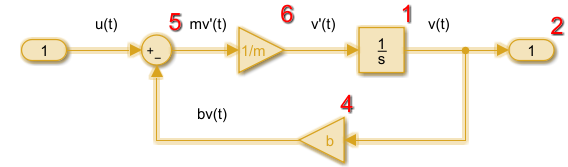（4）Simulation
Simulation is the process after model compilation where block method outputs and states are computed at successive time steps over a specified time range using a numerical solver.

During each simulation loop, Simulink calculates a Δt to determine the time step t(k+1) = t(k) + Δt. The size of Δt is based on an estimated error between the simulated solution and the actual solution. At the end of a simulation, data results are given as vectors [t, X, Y] for time, state and output at each time step.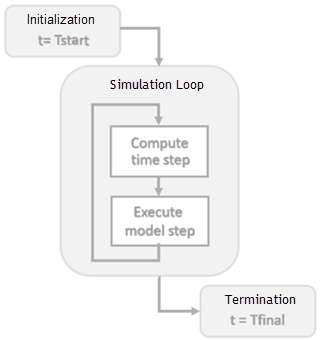（5）Solver
A Solver finds an approximate solution for a set of model equations. Simulink uses established numerical solvers for this task.

Solver step size can be fixed or variable:

• Fixed step – Time step T(k+1) = T(k) + Δt where Δt is constant. If step size is too large, simulation results can have a large error. In the following example, a step size of 2 distorts the shape of a sine wave signal. You can specify the size of the time step in the case of fixed-step solvers, or the solver can automatically determine the step size in the case of variable-step solvers.

• Variable step – Variable step solvers iterate to reach a solution based on an error tolerance. Time step T(k+1) = T(k) + Δtₖwhere Δtₖ changes from one simulation step to the next depending on the estimated error. Smaller time steps increase the accuracy of the simulation results. To minimize the computation workload, a variable-step solver chooses the largest step size consistent with achieving an overall level of precision specified by the error tolerance and observing zero-crossings. This ensures that all model states are computed to the accuracy specified by the user.

Choosing a solver method depends on the nature of the model equations. Euler’s method is a simple numerical solver that calculates the next value of y by using the slope (y') of a tangent line to y. If y is a function that integrates a ramp function x with a slope of 1, y' = x, and a numerical solver would use the following equations.

x[n+1] = x[n] + Δt*1
y[n+1] = y[n] + Δt*x[n+1]

Decreasing the step size increases the accuracy of the results. but it increases the time to complete a simulation. In the following example, a step size of 2 shows an error of about 20 percent after 10 seconds while a step size of 0.5 produces a result that is closer to the actual solution.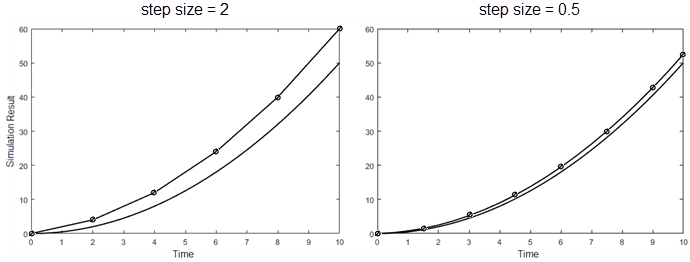# 9. Deep Learning Toolbox

Deep Learning Toolbox™ 提供了一个用于通过算法、预训练模型和 App 来设计和实现深度神经网络的框架。您可以使用卷积神经网络（ConvNet、CNN）和长短期记忆 (LSTM) 网络对图像、时序和文本数据执行分类和回归。您可以使用自动微分、自定义训练循环和共享权重来构建网络架构，如生成对抗网络 (GAN) 和孪生网络。使用 Deep Network Designer，您能够以图形方式设计、分析和训练网络。Experiment Manager 可帮助您管理多个深度学习试验，跟踪训练参数，分析结果，并比较不同试验的代码。您可以可视化层激活，并以图形方式监控训练进度。

## 9.2. 时序、序列和文本深度学习

10-087万+
01-152万+
05-2910万+
11-23185
01-142万+
01-301万+
03-01876
06-211万+
07-131万+
11-09554
12-097889
07-251837
11-202206
12-044621
11-07117
12-03138
03-092549
04-29877
01-09362

### “相关推荐”对你有帮助么？

•非常没帮助
•没帮助
•一般
•有帮助
•非常有帮助

©️2022 CSDN 皮肤主题：书香水墨 设计师：CSDN官方博客被折叠的  条评论 为什么被折叠?到【灌水乐园】发言¥2 ¥4 ¥6 ¥10 ¥20余额支付 (余额：-- )扫码支付获取中扫码支付点击重新获取扫码支付1.余额是钱包充值的虚拟货币，按照1:1的比例进行支付金额的抵扣。
2.余额无法直接购买下载，可以购买VIP、C币套餐、付费专栏及课程。余额充值